# 4.14 Current Mirror BJTs

### Bipolar Junction Transistor or BJT Current Mirror

An often-used circuit applying the bipolar junction transistor is the so-called current mirror, which serves as a simple current regulator, supplying nearly constant current to a load over a wide range of load resistances.

We know that in a transistor operating in its active mode, the collector current is equal to base current multiplied by the ratio β. We also know that the ratio between collector current and emitter current is called α. Because collector current is equal to base current multiplied by β, and emitter current is the sum of the base and collector currents, α should be mathematically derivable from β. If you do the algebra, you’ll find that α = β/(β+1) for any transistor.

We’ve seen already how maintaining a constant base current through an active transistor result in the regulation of collector current, according to the β ratio. Well, the α ratio works similarly: if emitter current is held constant, collector current will remain at a stable, regulated value so long as the transistor has enough collector-to-emitter voltage drop to maintain it in its active mode. Therefore, if we have a way of holding an emitter current constant through a transistor, the transistor will work to regulate collector current at a constant value.

Remember that the base-emitter junction of a BJT is nothing more than a PN junction, just like a diode, and that the “diode equation” specifies how much current will go through a PN junction given forward voltage drop and junction temperature:

Diode Equation Formula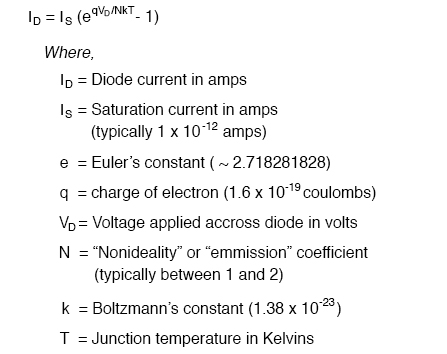If both junction voltage and temperature are held constant, then the PN junction current will be constant. Following this rationale, if we were to hold the base-emitter voltage of a transistor constant, then its emitter current will be constant, given a constant temperature. Consider the example figure below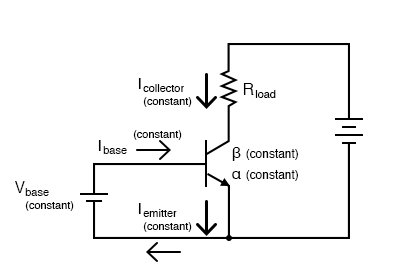Constant VBE gives constant IB, constant IE, and constant IC.

This constant emitter current, multiplied by a constant α ratio, gives a constant collector current through Rload if enough battery voltage is available to keep the transistor in its active mode for any change in Rload‘s resistance.

To maintain a constant voltage across the transistor’s base-emitter junction use a forward-biased diode to establish a constant voltage of approximately 0.7 volts, and connect it in parallel with the base-emitter junction as in Figure below.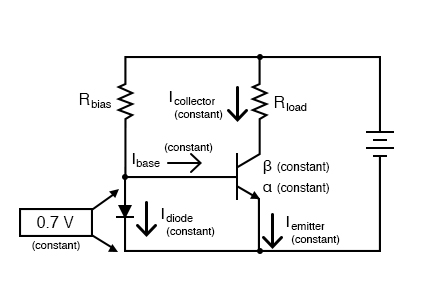Diode junction 0.7 V maintains constant base voltage, and constant base current.

The voltage drop across the diode probably won’t be 0.7 volts exactly. The exact amount of forward voltage dropped across it depends on the current through the diode, and the diode’s temperature, all in accordance with the diode equation. If diode current is increased (say, by reducing the resistance of Rbias), its voltage drop will increase slightly, increasing the voltage drop across the transistor’s base-emitter junction, which will increase the emitter current by the same proportion, assuming the diode’s PN junction and the transistor’s base-emitter junction are well-matched to each other. In other words, transistor emitter current will closely equal diode current at any given time. If you change the diode current by changing the resistance value of Rbias, then the transistor’s emitter current will follow suit, because the emitter current is described by the same equation as the diode’s, and both PN junctions experience the same voltage drop.

Remember, the transistor’s collector current is almost equal to its emitter current, as the α ratio of a typical transistor is almost unity (1). If we have control over the transistor’s emitter current by setting diode current with a simple resistor adjustment, then we likewise have control over the transistor’s collector current. In other words, collector current mimics, or mirrors, diode current.

Current through resistor Rload is, therefore, a function of the current set by the bias resistor, the two being nearly equal. This is the function of the current mirror circuit: to regulate current through the load resistor by conveniently adjusting the value of Rbias. Current through the diode is described by a simple equation: power supply voltage minus diode voltage (almost a constant value), divided by the resistance of Rbias.

To better match the characteristics of the two PN junctions (the diode junction and the transistor base-emitter junction), a transistor may be used in place of a regular diode, as in Figure below (a).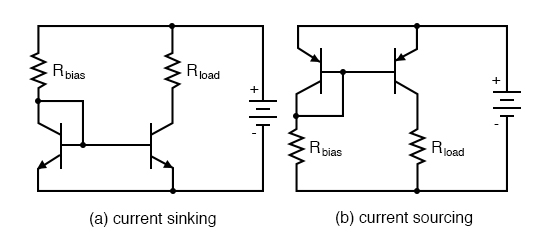Current mirror circuits.

Because temperature is a factor in the “diode equation,” and we want the two PN junctions to behave identically under all operating conditions, we should maintain the two transistors at exactly the same temperature. This is easily done using discrete components by gluing the two-transistor cases back-to-back. If the transistors are manufactured together on a single chip of silicon (as a so-called integrated circuit, or IC), the designers should locate the two transistors close to one another to facilitate heat transfer between them.

The current mirror circuit shown with two NPN transistors in the figure above (a) is sometimes called a current-sinking type because the regulating transistor draws the current from the load to ground (“sinking” current), rather than forcing it to flow from the positive side of the battery to the load (“sourcing” current). If we wish to have a grounded load, and a current sourcing mirror circuit, we may use PNP transistors like Figure above (b).

While resistors can be manufactured in ICs, it is easier to fabricate transistors. IC designers avoid some resistors by replacing load resistors with current sources. A circuit like an operational amplifier built from discrete components will have a few transistors and many resistors. An integrated circuit version will have many transistors and a few resistors. In Figure below One voltage reference, Q1 drives multiple current sources: Q2, Q3, and Q4. If Q2 and Q3 are equal-area transistors the load currents Iload will be equal. If we need a 2·Iload, parallel Q2 and Q3. Better yet fabricate one transistor, say Q3 with twice the area of Q2. Current I3 will then be twice I2. In other words, load current scales with the transistor area.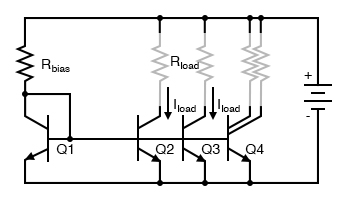Multiple current mirrors may be slaved from a single (Q1 – Rbias) voltage source.

Note that it is customary to draw the base voltage line right through the transistor symbols for multiple current mirrors! Or in the case of Q4 in the figure above, two current sources are associated with a single transistor symbol. The load resistors are drawn almost invisible to emphasize the fact that these do not exist in most cases. The load is often another (multiple) transistor circuit, say a pair of emitters of a differential amplifier, for example, Q3 and Q4 in “A simple operational amplifier”,Ch 8. Often, the collector load of a transistor is not a resistor but a current mirror. For example the collector load of Q4 collector, Ch 8 is a current mirror (Q2).

For an example of a current mirror with multiple collector, outputs see Q13 in the model 741 op-amp, Ch 8. The Q13 current mirror outputs substitute for resistors as collector loads for Q15 and Q17. We see from these examples that current mirrors are preferred as loads over resistors in integrated circuitry.

REVIEW:

• A current mirror is a transistor circuit that regulates the current through a load resistance, the regulation point is set by a simple resistor adjustment.
• Transistors in a current mirror circuit must be maintained at the same temperature for precise operation. When using discrete transistors, you may glue their cases together to do this.
• Current mirror circuits may be found in two basic varieties: the current sinking configuration, where the regulating transistor connects the load to the ground; and the current sourcing configuration, where the regulating transistor connects the load to the positive terminal of the DC power supply.

Back to Main Index of Book